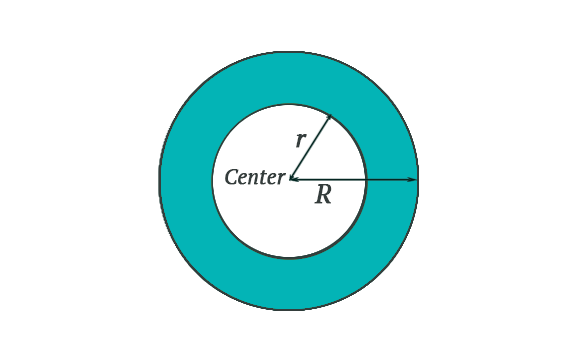# Annulus Formula

An Annulus is a ring-shaped object, bounded by the circumference of two concentric circles of two different radii. An Annulus is much like the throw-ring. One way to think of it is a circular disk with a circular hole in it. The outer and inner circles that define the ring are concentric, that shares a common center point.The dimensions of an annulus are defined by the two radii R, r, which are the radii of the outer ring and the inner ‘hole’ respectively. The area of a circular ring can be found by subtraction the area of a small circle from that of the large circle.

Here are formulas to find Area of Annulus.

\begin{array}{l}\begin{aligned} A &=\pi\left(R^{2}-r^{2}\right) \\ R &=\sqrt{r^{2}+\frac{A}{\pi}} \\ r &=\sqrt{R^{2}-\frac{A}{\pi}} \end{aligned}\end{array}

Where,
A = Area of Annulus
(Pi)

$$\begin{array}{l}\pi\end{array}$$
= is approximately 3.142

### Solved Example

Example : Find the area of the path, where a path is 14 cm wide, surrounds a circular lawn whose diameter is 360 cm.
Solution:
Given,

Width of the path = 14 cm
Diameter of the inner circle is 360 cm.

Radius of inner circle (r) = 360/2 = 180 cm
Radius of outer circle is (R) = 180 + 14 = 194 cm

A =

$$\begin{array}{l}\pi\end{array}$$
(
$$\begin{array}{l}R^{2}\end{array}$$
$$\begin{array}{l}r^{2}\end{array}$$
)
= 3.142 (R + r)(R – r)
= 3.142 (194 + 180) (194 – 180)
= 3.142
$$\begin{array}{l}\times\end{array}$$
374
$$\begin{array}{l}\times\end{array}$$
14
= 16451.512 cm2